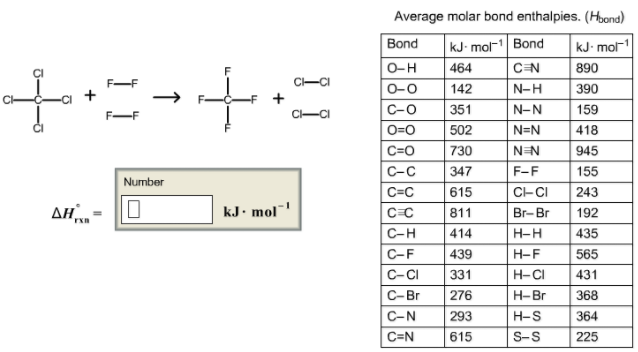# Problem: Use the molar bond enthalpy data in the table to estimate the value of ΔH°rxn for the equationCCI4(g) + 2F2(g) → CF4(g) + 2CI2(g)The bonding in the molecules is shown here.

###### FREE Expert Solution
94% (216 ratings)
###### Problem Details

Use the molar bond enthalpy data in the table to estimate the value of ΔH°rxn for the equation

CCI4(g) + 2F2(g) → CF4(g) + 2CI2(g)

The bonding in the molecules is shown here.What scientific concept do you need to know in order to solve this problem?

Our tutors have indicated that to solve this problem you will need to apply the Bond Energy concept. If you need more Bond Energy practice, you can also practice Bond Energy practice problems.

What is the difficulty of this problem?

Our tutors rated the difficulty ofUse the molar bond enthalpy data in the table to estimate th...as medium difficulty.

How long does this problem take to solve?

Our expert Chemistry tutor, Dasha took 4 minutes and 38 seconds to solve this problem. You can follow their steps in the video explanation above.

What professor is this problem relevant for?

Based on our data, we think this problem is relevant for Professor Kobko-Litskevitch's class at HUNTER.